### Discharge Characteristics of Ogee Crest Uncontrolled Flow

For an ogee crested control weir for a spillway without any control with a gate, the free flow discharge equation is given as

Where Q is the discharge (in m3/s), Cd is the coefficient of discharge, Le is the effective length of crest (in m), including velocity of approach head. The discharge coefficient, Cd, is influenced by a number of factors, such as:

1. Depth of approach

2. Relation of the actual crest shape to the ideal nappe shape

3. Upstream face slope

4. Downstream apron interference, andDownstream submergence

The effect of the above mentioned factors on the variation of discharge and calculation for effective length are mentioned in the following paragraphs. Effect of depth of approach :

• For a high sharp-crested ogee shaped weir, as that of a Overfall spillway of a large dam, the velocity of approach is small and the lower nappe flowing over the weir attains maximum vertical contraction. As the approach depth is decreased, the velocity of approach increases and the vertical contraction diminishes. For sharp-crested weirs whose heights are not less than about one-fifth of the head producing the flow, the coefficient of discharge remains fairly constant with a value of about 1.82 although the contraction diminishes. For weir heights less than about one-fifth the head, the contraction of the flow becomes increasingly suppressed and the crest coefficient decreases. This is the case of an ogee crested chute spillway control section. When the weir height becomes zero, the contraction is entirely suppressed and the weir turns into a broad crested one, for which the theoretical coefficient of discharge is 1.70. The relationship of the ogee crest coefficient of discharge Cd for various values of P/Hd where P is the height of the weir above base and Hd is the design head, is given in Figure 25. The coefficients are valid only when ogee is formed to the ideal nappe shape.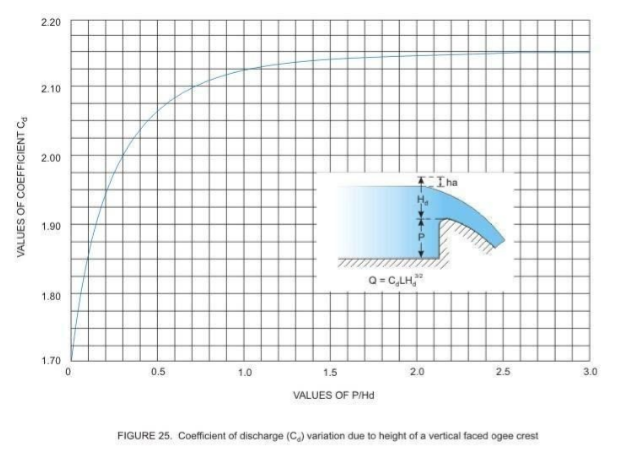Effect of The Crest Shape Differing From The Ideal Nappe Shape

• When the ogee crest is formed to a shape differing from the ideal nappe shape  or when the crest has been shaped for a head larger or smaller than the one under consideration, the coefficient of discharge will differ from that given in the previous section. A wider crest shape will reduce the coefficient of discharge while a narrower Crest Shape will reduce the coefficient. The application of this concept is required to deduce the discharge flowing over a spillway when the  flow is less or more than the design discharge. The variation of the coefficient of discharge in relation to H/Hd, where H is the actual head and Hd is the design head, is shown in Figure 26.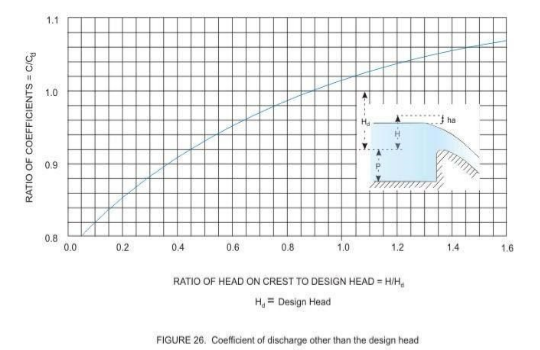Effect of Upstream Face Slope

• For small ratio of P/Hd where P is the height of the weir and Hd the design head, as for the approach to a chute spillway, increase of the slope of upstream face tends to increase the coefficient of discharge, as shown in Figure 27. This figure shows the ratio of the coefficient for ogee crest with a sloping face to that with vertical face. For large ratios of P/Hd, the effect is a decrease of the coefficient. The coefficient of discharge is reduced for large ratios P/Hd only for relatively flat upstream slopes.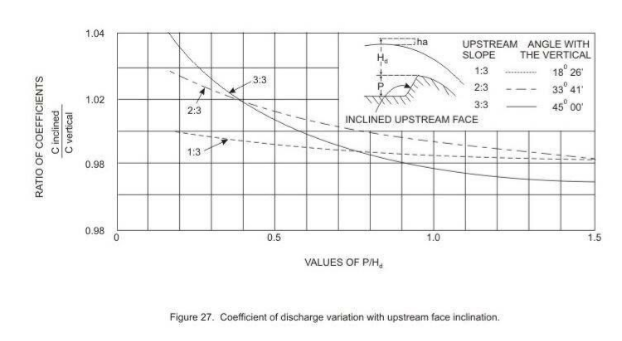Effect of Downstream Apron Interference And Downstream Submergence

• This condition is possible for dams of relatively small heights compared to the natural depth of the river, when the water level downstream of the weir crest is high enough to affect the discharge, the condition being termed as submerged. The conditions that after the coefficient of discharge in this case are the vertical distance from the crest of the over flow to the downstream apron and the depth  of flow in the downstream channel, measured above the apron.

Five distinct characteristic flow conditions can occur below an overflow crest, depending on the relative positions of the apron and the downstream water surface:

1. The flow will continue at supercritical stage

2. A partial or incomplete hydraulic jump will occur immediately downstream  from the crest

3. A true hydraulic jump will occur

4. A drowned jump will occur in which the high-velocity jet will follow the face of the overflow and then continue in an erratic and fluctuating path for a considerable distance under and through the slower water, and

5. No jump will occur - the jet will break away from the face of the overflow and ride along the surface for a short distance and then erratically intermingle with the slow moving water underneath.

• According to USBR (1987), the relationship of the floor positions and downstream submergences which produce these distinctive flows can be shown in a graph as in Figure 28.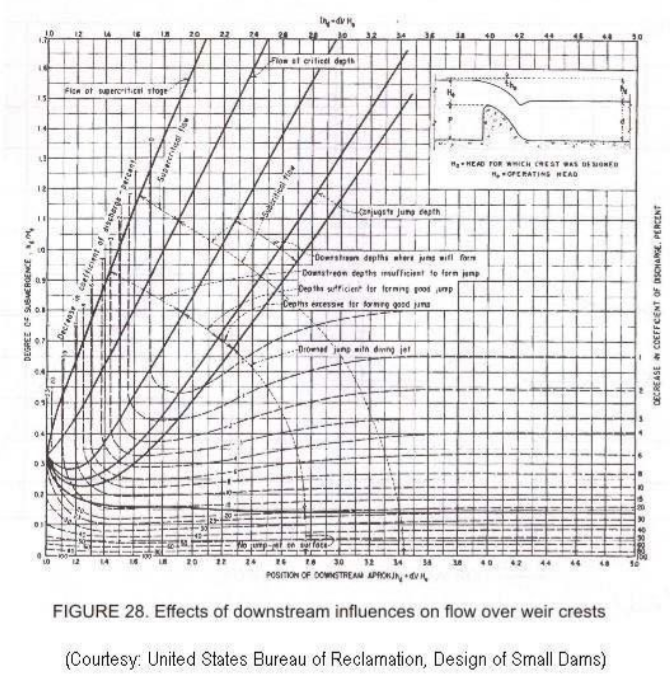• Usually for large dams the cases A,B or C with dominate and the decrease in the coefficient of discharge is due principally to the back pressure effect of the downstream apron and is independent of any submergence effect due to tail water. Cases D and E can be expected t o be found in low-height dams like small height diversion or  navigation dam. Figure 29, adapted from USBR (1987), shows the effect of downstream apron conditions on the coefficient of discharge. It may be noted that this curve plots the same data represented by the vertical dashed lines of Figure 28 in a slightly different form.  As  the  downstream  apron  level  nears  the  crest  of  the  overflow ( hd  de approaches 1.0), where hd is the difference of total energy on upstream and the water level downstream, d is the downstream water depth and He is the total energy upstream measured above the crest of the weir, the coefficient of discharge is about 77 percent of hd   that for un-retarded flow. From Figure 29 it can be seen that when the ratio of d values exceeds about 1.7, the downstream floor position has little effect on the coefficient, but there is a decrease in the coefficient caused by tail water submergence.

• Figure 30 shows the ratios of the coefficient of discharge where affected by tailwater conditions, to that coefficient for free flow conditions. This curve plots the data represented by the horizontal dashed lines on Figure 28 in a slightly different form. Where the dashed lines of Figure 28 are curved, the decrease in the coefficient is the result of a combination of tail-water effects and downstream apron position.

​​​​​​​​​​​​​​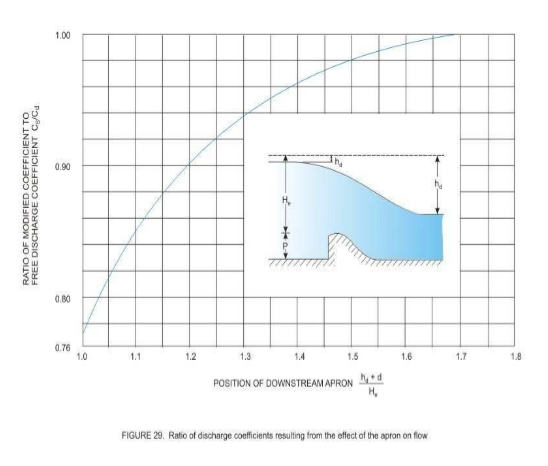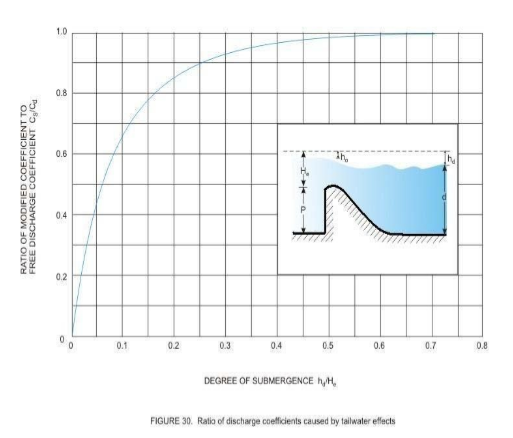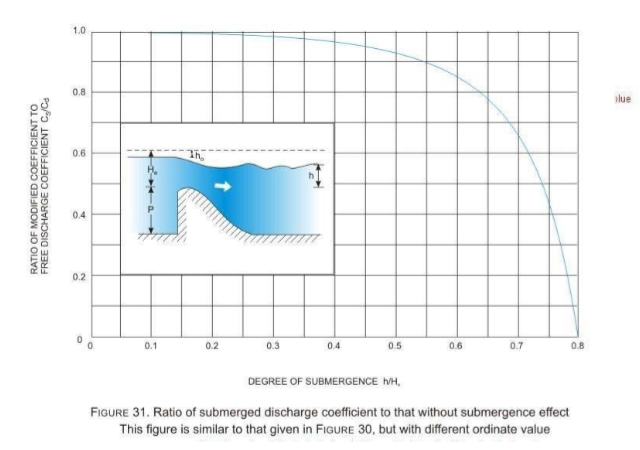• The total head on the crest He, does not include allowances for approach channel friction losses due to curvature into the inlet section, and inlet or transition losses. Where the design of the approach channel results in appreciable losses, they must be added to He to determine reservoir elevations corresponding to the discharges given by the discharge equation.

• Ii

• Where the crest piers and abutments are shaped to cause side contractions of the overflow, the effective length, Le, will be the net length of the crest, L. The effect of the end contractions may be taken into account by reducing the net length of crest as given below:
Le L 2N p a e

• Where L, Le and H have been explained before, N is the number of piers and Kp and Ka are the pier and abutment contraction coefficients. The reason for the reduction of the net length may be appreciated from Figure 32.

​​​​​​​​​​​​​​​​​​​​​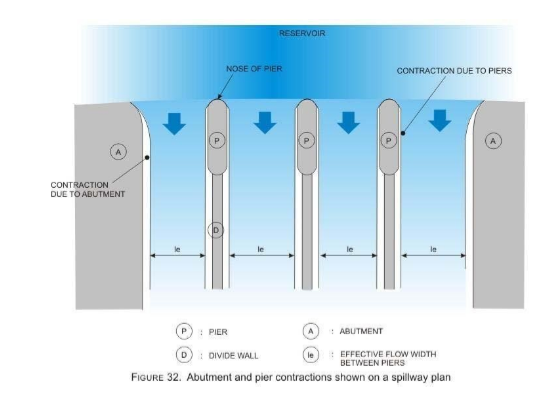The pier contraction coefficient Kp depends upon the following factors:

• Shape and location of the pier nose

• Thickness of the pier

• Approach velocity

​​​​​​​For the condition of flow at the design head, the average values of pier contraction coefficients may be assumed as shown in Figure 33.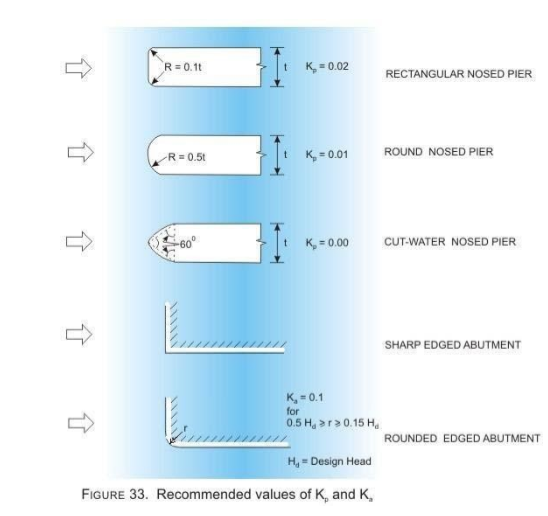The abutment contraction coefficient is seen to depend upon the following factors:

1. Shape of abutment

2.  Angle between upstream approach wall and the axis of flow